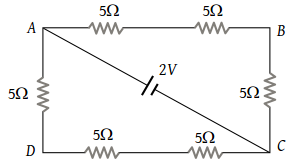The potential difference between points A and B of adjoining figure is(1) $\frac{2}{3}V$

(2) $\frac{8}{9}V$

(3) $\frac{4}{3}V$

(4) 2 V